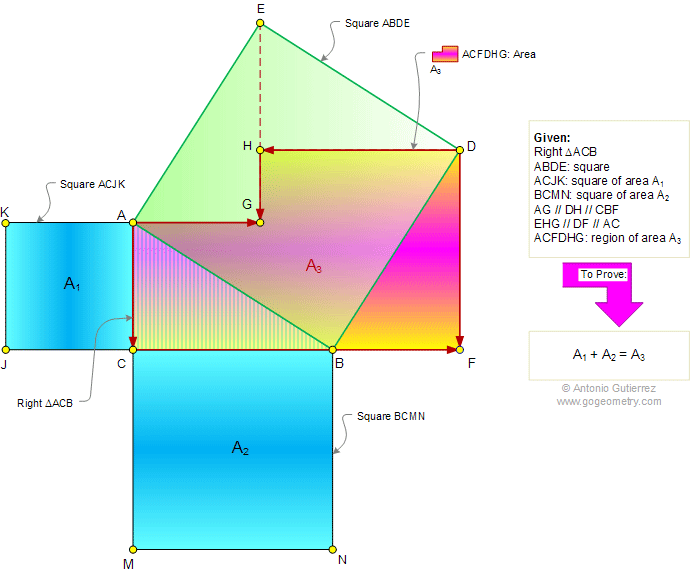# Problem 540: Right Triangle, Square, Parallel, Perpendicular, Dissection, Area, Pythagoras. Level: High School, SAT Prep, College

 The figure shows a right triangle ACB with the squares ABDE, ACJK (area A1), and BCMN (area A2). AG and DH are parallel to line CBF. Line EHG and DF are parallel to AC. If A3 is the area of the region ACFDHG, prove that A1 + A2 = A3. Post a comment or solution.Home | Search | Geometry | Problems | All Problems | Pythagoras | Area of a Triangle | Right Triangle Area | Geoboard | Email | By Antonio Gutierrez